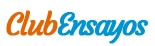# The Economies of Scale and Scope

Enviado por   •  17 de Mayo de 2013  •  1.504 Palabras (7 Páginas)  •  302 Visitas

Página 1 de 7

The Economies of

Scale and Scope

Jose Carlos Brioso Fernández

B00599400

INdex

Economies and Diseconomies of Scale 3

Long-Run Average Cost 3

Minimum Efficient Scale 4

Economies of Scale and Natural Monopoly 5

Examples of Economies of Scale 5

Economies of Scope 6

Examples of Economies of Scope 6

Economies of Scale and Scope Related with the Firm’ Size 6

Examples 7

Bibliography 8

The economies of scale and scope are advantages that the firms can obtain to reduce their average unit cost. The firsts are achieved when the firm increase the produced amount. The seconds are obtained then the firm expanded its product range.

Economies and Diseconomies of Scale

The economies of scale occur when the average cost of a good decrease to produce more quantity of this good. Other way to explain is when the cost of producing a unit of a good falls as its output rates increases.

The economies of scale usually happen when the variable cost is considerably less than the fixed cost. So the fixed cost can be more divided when there are more units produced. It can be because the machinery or the technology is very expensive. Another way to achieve economies of scale is when the price of the inputs decrease when their use increase or when another production plant is added to the company (more than one).

In the case of the assembly line production is a production process which needs a great initial investment (fix cost), but when a lot of goods are produced the average cost decrease with each unit produced.

There are two types of economies of scale:

Internal economies of scale: the cost per unit depends on size of the individual firm. For example, an improvement in business efficiency.

External economies of scale: the cost per unit depends on the size of the industry. For example, an improvement in transports.

But increasing production is not always beneficial. When the firm grows so large often happens that the cost per unit increase. Some of the reasons why it occurs are the difficult and expensive management of a big business, the cost of communicating both up and down the management hierarchy... This phenomenon is called diseconomy of scale.

The diseconomy of scale is the opposite of the economy of scale. It occurs when the average cost of producing a good increases when the units produced increase too.

Long-Run Average Cost

The long-run average cost is a business metric that represent the average cost per unit of output over the long-run, where all inputs are considered to be variable. The inputs are considered variable because in the long-run the companies have the flexibility to change big components of their operations to achieve optimal efficiency.

In the long-run average cost curve:

The economies of scale are present when the LRAC (Long-Run Average Cost) curve slopes downward.

The diseconomies of scale are present when the LRAC curves slope upward.

This graphic simulates a firm which incorporates more factories in its plant (long-run). Each AC curve is the average cost of a factory and the blue curve is the total average cost. The first two factories generate economies of scale but when the firm decides to increase its plant with another factory, the diseconomies of scale begin to appear.

Return to scale express how varies the amount produced by a company as it varies the use of all the inputs involved in the production process in the same proportion (ratio input-output). There are increasing, constant and decreasing returns to scale:

Increasing returns to scale: when the variation of inputs is smaller than the variation outputs (proportionally).

Constant returns to scale: when varying the proportion of inputs produce the same variation in the proportion of outputs.

Decreasing returns to scale: when the variation of inputs is bigger than the variation outputs (proportionally).

Minimum Efficient Scale

The minimum efficient scale is the smallest amount of production that a company can achieve using economies of scale. It is the optimal situation for a firm. From this point diseconomies of scale begins.

In this graph Q is the minimum efficient scale.

Economies of Scale and Natural Monopoly

When diseconomies of scale don’t appear, the natural monopoly occurs. It happens when the average costs decrease continuously. The marginal cost (the cost of the additional inputs needed to produce that output) always decrease.

This fact makes the most efficient way to cover the demand is just one company. It means that there are a high capital costs relative to variable costs and the market size generating barriers to entry for other companies. It is economically non-viable.

In this graph the LRAC always decrease.

For an infinite amount of production the average cost would tend to zero.

For any shift in the demand curve will always be efficient

...

Descargar como (para miembros actualizados)  txt (9.8 Kb)
Leer 6 páginas más »
Disponible sólo en Clubensayos.com